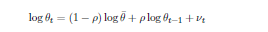# Impossible to find the steady state in rbc model with labour shock (large sum of residuals)

I am new to Dynare and I am trying to solve rbc model with labour supply shock. Yet, it says to me that is impossible to find steady state. May you probably suggest or give me a clue what is wrong. Here is my code:
// rbc model with labour supply shock
var c i n k y theta;

varexo eps;

parameters beta alpha delta rho thetas;

ks = 944556
ys = 207171
kys = 4.5593;
iys = 0.2576;
alpha = 0.3842;
ns = 0.4614;
cys = 1-iys;
rho = 0.9;
cs = cysys;
is = iys
ys;

thetas = (1-alpha)*ys / (cs *(ns^2));

// get a value for delta from eq involving depreciation rate
delta = iys / kys;

// knowing delta we can get betta

beta = 1/((alpha/kys)- delta +1);

model;
// 1. (n) Labor market
n = (1 - alpha)y/(nthetac);
// 2. © Consumption Euler equation.
1/c = beta
(alphay(+1)/k+1-delta)/c(+1);
// 3. (k) Resource constraint
y = 1
(k(-1)^alpha)*(n^(1-alpha));
// 4. (i) Investment
i = k-(1-delta)k(-1);
// 5. (y) GDP
y = c+i;
// 6. (z) labor supply shock
theta = (thetas^(1-rho))
( theta(-1)^rho)*exp(eps);
end;

initval;
c = cs;
i = is;
k = ks;
y = ys;
n = ns;
theta = 1;
end;

check;

shocks;
var eps = 0.01^2;
end;

stoch_simul(order=1,periods=500,loglinear);
// extended_path(periods=500);
pause;
close;

plot(y-log(ys))
pause;
close;

Error using print_info (line 32)
Impossible to find the steady state (the sum of square residuals of the static equations is 42869001355.2616). Either the
model doesn’t have a steady state, there are an infinity of steady states, or the guess values are too far from the
solutionrbc_practice.mod (1.1 KB)

Why do you have such strange steady state initial values? And what is the logic behind

thetas = (1-alpha)*ys / (cs *(ns^2));


Why not simply 1 ?

@jpfeifer , because my labour supply shock looks this wayso I need this thetas to model this shock, and I get it out of labour market equilibrium

Having the log-process is fine. The question is where the exact value for \bar \theta comes from.